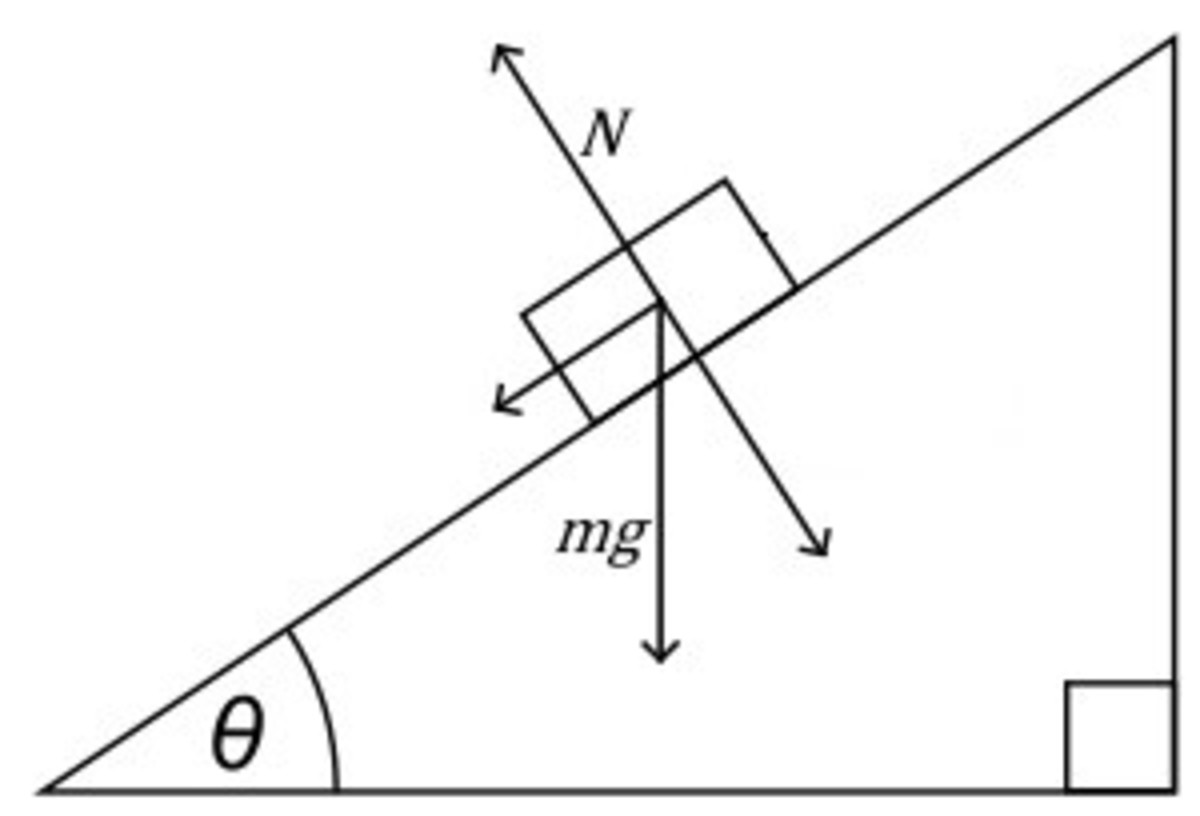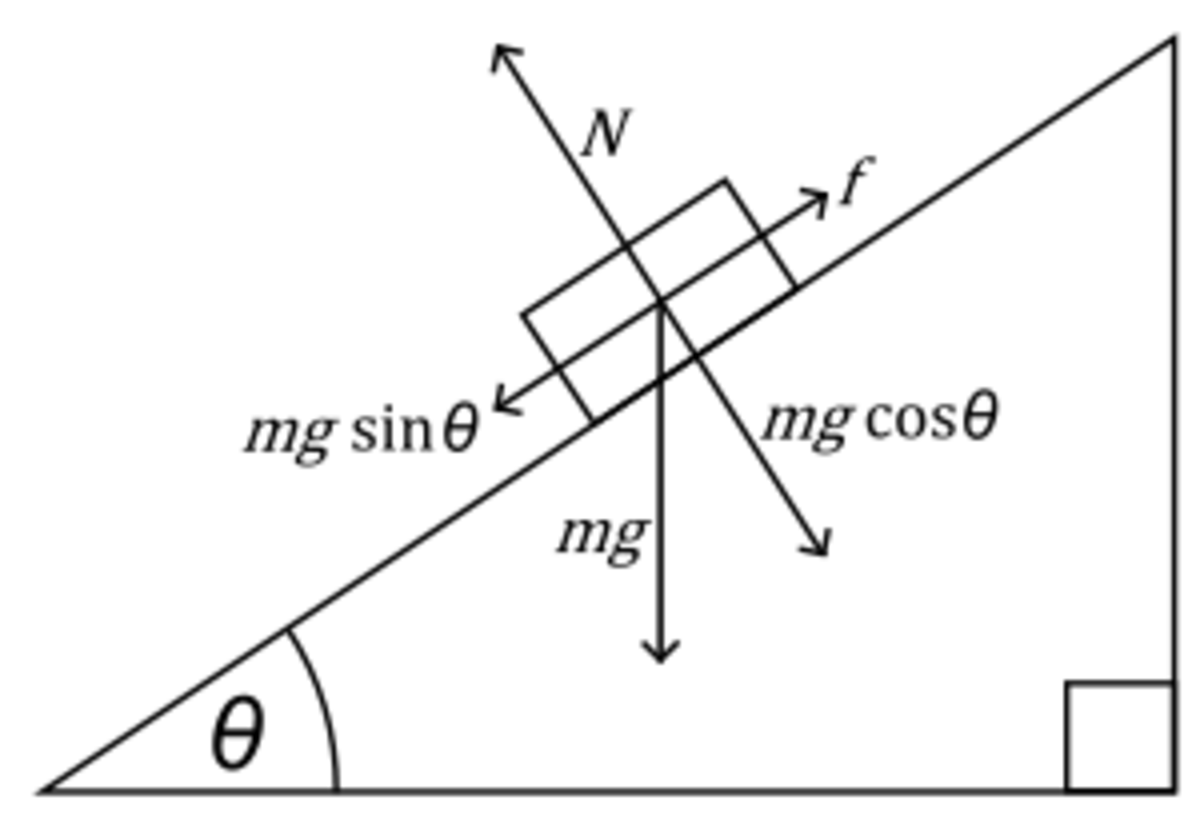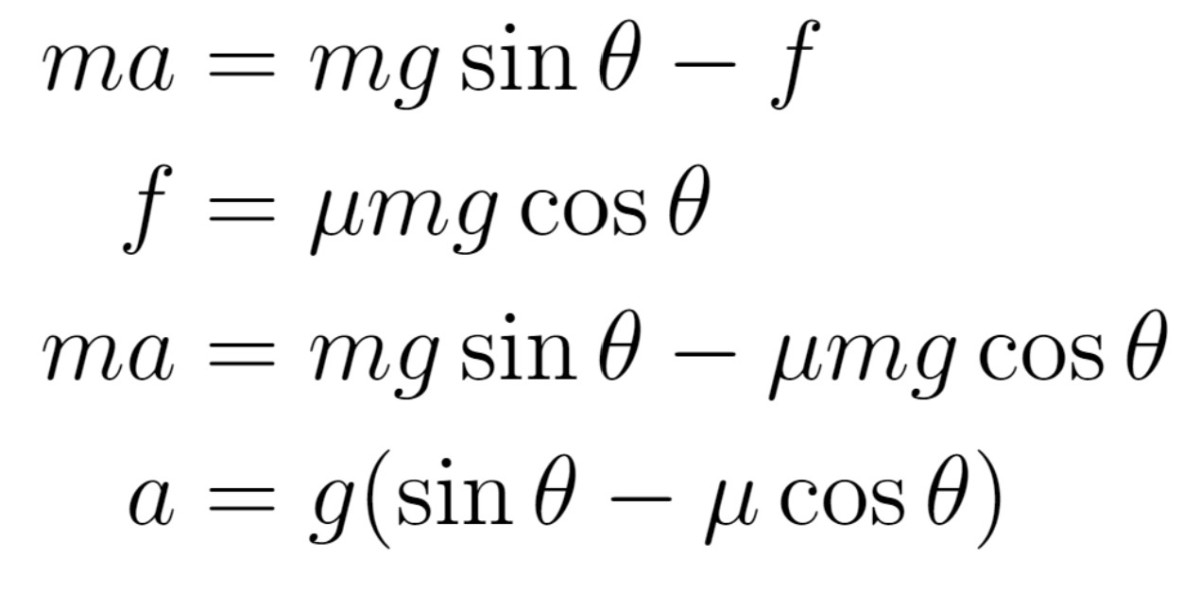# Basic Mechanics: Motion on an Inclined Plane

Mohammad Yasir is a physics graduate from the University of Delhi and currently enrolled in the master's programme at IIT.

## Introduction

An inclined plane is a flat surface that is raised on one end and thus, "inclined" or tilted at an angle with respect to the ground. They come in particularly handy for transporting heavy objects to a raised surface since it would naturally be easier to slide heavy objects on a plane than lift them over a flight of stairs.

However, we are concerned with a physicist's view of the inclined plane, which means that by the end of this article, you'll be able to explain all the forces an object on an inclined plane feels. What's more, you'll also be able to calculate the magnitudes of these forces, given a set of initial values.

## How do we Tackle Inclined Plane Motion?

To the uninitiated, inclined plane motion can feel like a nightmare. Worry not, though. As someone who's been playing around with the concepts of physics for the past seven years, I can confirm that with the proper knowledge, it is more a peaceful dream than a torrid nightmare. That said, I do advise you not to let overconfidence or a rushed calculation ruin your solution.

To tackle inclined plane motion, you must first have a problem statement at hand. To make things simpler, I will start with a situation where frictional forces can be ignored. As we will soon see, the opposite scenario will then be a quite simple matter of adding a couple of terms. For now, here is your problem statement:

Calculate the acceleration of an object of mass m, sliding down an inclined plane of angle θ. Consider the inclined plane to be smooth and thus, frictional forces to be zero.

In the image I attached above, let us try to figure out the forces acting on the mass m. These will be:

1. The gravitational force mg, acting downwards on the mass m.
2. A component of gravitational force parallel to the inclined plane.
3. A component of gravitational force perpendicular to the inclined plane.
4. The normal reaction exerted by the inclined plane on the mass.The mass m will experience gravitational force mg downwards. There will be two components of this force, one parallel and one perpendicular to the incline. Finally, we'll have a normal reaction N on the mass by the incline.

## Calculating the Components

As you can see in the initial diagram, the object is moving in a direction parallel to the inclined plane. Which means our actual job is to find the acceleration (or equivalently, the force) acting in this direction. Naturally, since there are no external forces, this force will be calculated in terms of the components of gravitational force.

To that end, here is a little bit of construction I did on our drawing.

In this figure, I have drawn a normal on OB, and represented it by AD. This makes the ∠BAD = 90º. Further, since AC is the direction of the gravitational force, it is already normal to OE. Now, take a look at the following calculations.

Scroll to Continue

## Complicated Much?

The calculations above might seem daunting at first. But let me remind you to focus on the outcome. We have succeeded, without even diving into any particulars of the problem, in proving that ∠CAD = θ. This serves two purposes:

1. Regardless the problem statement, we have proved that the angle between the vertical and the normal on the inclined plane is equal to the angle of the incline itself. We can reuse this result in all inclined plane problems.
2. Since we now have the value of ∠CAD, we can now find the components of gravitational force experienced by the mass m.

Indeed, if you recall vector resolution, you can easily see that the perpendicular and parallel components of the gravitational force on our mass m can be written as follows:

## So, What Next?

As far as our initial problem statement goes, we have already done what we set out to do. The problem asked us what the acceleration of an object sliding down a smooth incline would be and presto! We got it.

What we calculated above was a highly idealistic scenario where frictional losses could be ignored. But what if the inclined plane weren't smooth? As it turns out, that is also quite simple. In fact, let us rephrase our problem statement and ask this:

Calculate the acceleration of an object of mass ﻿m﻿, sliding an inclined plane with coefficient of friction μ and inclination θ.

As usual, the first step is to draw a free body diagram and represent the forces acting on it. Since the object tends to slide down the incline, the direction of frictional force is up the incline.This time, we have an additional frictional force f, acting on m upwards along the incline.

Before we can continue, we must find the magnitude of this frictional force. Concepts from mechanics have already told us that frictional force f = μN, where N is the normal reaction. Thus, our job is to find N. And that is all too easy using Newton's second law. Equating forces perpendicular to the incline to zero gives us the following result:

With that done, all that is left to do is apply the second law once more. Only this time around, we'll apply it parallel to the inclined plane. Considering acceleration towards the bottom of the incline plane to be positive, we have:Using Newton's second law, we have been able to find the acceleration of the mass m.

## And We're Done

There we have it. The above equation for acceleration is a general equation of motion for an object sliding down a rough inclined plane of inclination θ and with coefficient of friction μ. In case the inclined plane is smooth, we can simply substitute μ = 0 and arrive at the result we obtained earlier.

Despite the lengthy nature of this article, the concept of inclined plane motion is quite easy to handle. In fact, the sole reason I let this article go this long is because I wanted to tackle the problem in detail. However, as you can see, the final result is worth the effort. We have ourselves, a beautiful equation that you can use to solve inclined plane motion regardless of frictional losses.

To conclude, here is a summary of how to solve problems like these:

1. Draw the free body diagram.
2. Resolve forces along directions that can prove useful. For example, when there is circular motion, resolve forces along and perpendicular to the radius.
3. Apply Newton's second law in each direction to equate the acceleration of the mass with the resultant forces. This will give us the equation of motion.
4. Use the equation of motion obtained above to calculate further if required.

This content is accurate and true to the best of the author’s knowledge and is not meant to substitute for formal and individualized advice from a qualified professional.